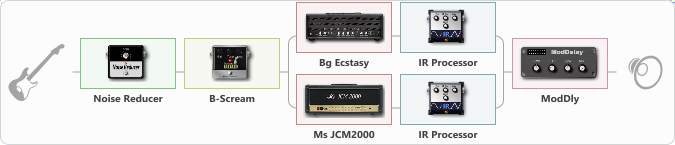Discussion in 'ToneLib-GFX presets' started by carlinos3, May 31, 2021.

Effects chain:Effect: "Noise Reducer" (Dinámica / Filtro), active - "yes"
{
"Sens" = 61
"Mode" = Hard
}

Effect: "B-Scream" (Overdrive / Distorsión), active - "yes"
{
"Drive" = 37
"Tone" = 75
"Level" = 100
}

Effect: "Splitter" (Dinámica / Filtro)
{
"A-Bypass" = Off
"A-Pan" = -61
"A-Level" = 55
"B-Bypass" = Off
"B-Pan" = 62
"B-Level" = 57

'A' branch:
{

{
"Gain" = 100
"Bass" = 83
"Middle" = 50
"Treble" = 50
"Presence" = 73
"Master" = 72
"Level (dB)" = 7
}

Effect: "IR Processor" (Gabinetes), active - "yes"
{
"IR" = am_dc
"Low Cut (Hz)" = 0
"Hi Cut (kHz)" = 20.0
"Mix" = 100
"Level (dB)" = 3
}
}
'B' branch:
{

{
"Gain" = 100
"Bass" = 76
"Middle" = 50
"Treble" = 50
"Presence" = 76
"Master" = 79
"Level (dB)" = 5
}

Effect: "IR Processor" (Gabinetes), active - "yes"
{
"IR" = charv.1_dc
"Low Cut (Hz)" = 0
"Hi Cut (kHz)" = 20.0
"Mix" = 100
"Level (dB)" = 2
}
}
}

Effect: "ModDly" (Retrasar), active - "yes"
{
"Time" = 508
"Feedback" = 23
"Tone" = 66
"Speed" = 1.7
"Mix" = 49
}

Note: You will need to download and install the ToneLib-GFX software to use the preset.

File size:
62.6 KB
Views:
2,542
2. ejemplo

File size:
1.3 MB
Views:
15